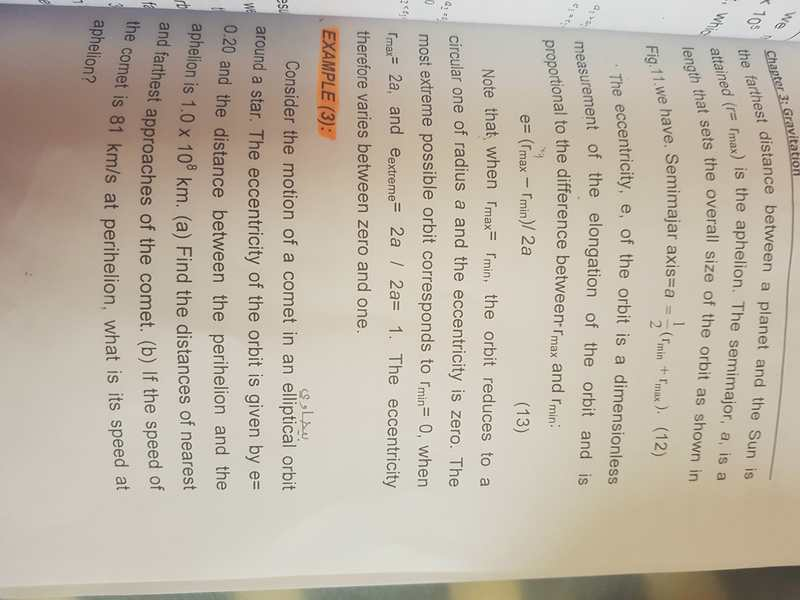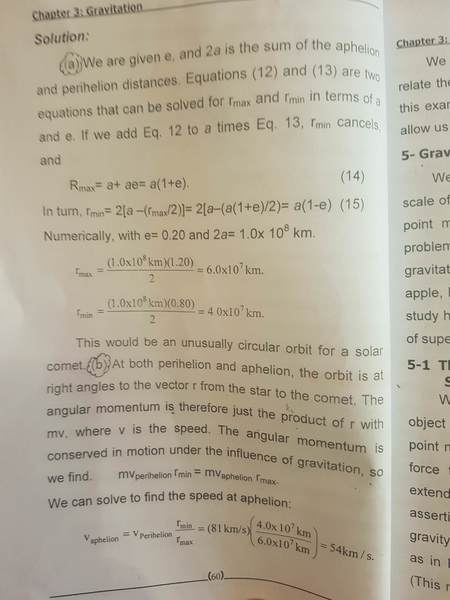# Example in chapter: Gravitation

EngMan

## Homework Statement

consider the motion of a comet in an elliptical orbit around a star. the eccentricity of the orbit is given by e= 0.20 and the distance between the perhelion and the aphelion is 1.0 × 10^power8

a- find the distances of nearest and farthest approaches of the comet.
b- if the speed of the comet is 81 km/s at perihelion, what is its speed at aphelion?

there is a solution but i cant understand it
i hope someone help me step by step## Answers and Replies

mfb
Mentor
Which part is unclear?

EngMan
Which part is unclear?
how Rmax=1×10^8 × 1.20 ÷ 2
is the second picture

mfb
Mentor
By definition, the eccentricity tells you how much periapsis and apoapsis deviate from the semi-major axis, here 20%=0.2. Periapsis is at (1-0.2) times the semi-major axis, and apoapsis is at (1+0.2) times the semi-major axis.

The semi-major axis is the mean of the two, and you are given the sum, so you have to divide the sum by 2 to get the the semi-major axis.